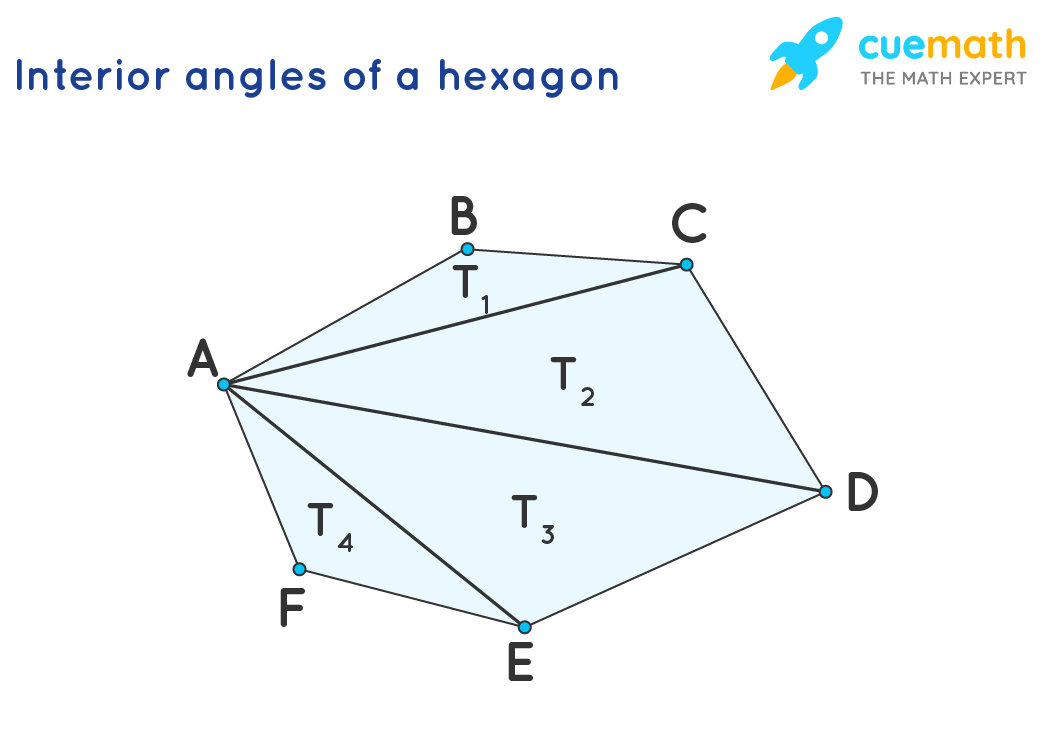# The Sum of The Interior Angles of a Hexagon

## Question: What is the sum of the interior angles of a hexagon?

hexagon is a polygon with six sides.

## Answer: The sum of the interior angles of a hexagon is 720°

An interior angle is an angle measured between the two sides of a polygon.

## Explanation:

The sum of the interior angles of an hexagon can be calculated in two ways:

• Dividing hexagon into triangles
• Interior angle sum formula

### Dividing hexagon into triangles

In this method, we are going to divide the hexagon into four triangles as shown belowThe hexagon is divided into four triangles namely △BCA, △CDA, △DEA, △EFA

The sum of the interior angles = ∠A + ∠B + ∠C + ∠D + ∠E + ∠F

= (∠BAC + ∠CAD + ∠DAE + ∠FAE ) + ∠B + (∠BCA + ∠DCA) + (∠CDA + ∠EDA) + (∠AEF + ∠AED) + ∠F

= (∠BAC + ∠B + ∠BCA) + (∠CAD + ∠DCA + ∠CDA ) + (∠EDA + ∠DAE + ∠AED)  + ( ∠AEF + ∠FAE + ∠F) (Rearranging the terms)

= Sum of interior angles of △BCA + Sum of interior angles of △CDA + Sum of interior angles of △DEA + Sum of interior angles of △EFA

= 180° + 180° + 180° + 180°  (Since, sum of interior angles of a triangle is 180°)

= 720°

### Interior angle sum formula:

Sum of interior angles of a Polygon = (n - 2) × 180°

where, n = number of sides

For a hexagon, n = 6

Thus, by substituting in the above formula we get

Sum of interior angles of a hexagon = (6 - 2) × 180° = 720°